# scikit-learn-k-means¶

Credits: Forked from PyCon 2015 Scikit-learn Tutorial by Jake VanderPlas

In :
%matplotlib inline
import numpy as np
import matplotlib.pyplot as plt
import seaborn;
from sklearn.linear_model import LinearRegression
from scipy import stats
import pylab as pl

seaborn.set()


## K-Means Clustering¶

In :
from sklearn import neighbors, datasets

X, y = iris.data, iris.target
from sklearn.decomposition import PCA
pca = PCA(n_components=2)
pca.fit(X)
X_reduced = pca.transform(X)
print("Reduced dataset shape:", X_reduced.shape)

import pylab as pl
pl.scatter(X_reduced[:, 0], X_reduced[:, 1], c=y,
cmap='RdYlBu')

print("Meaning of the 2 components:")
for component in pca.components_:
print(" + ".join("%.3f x %s" % (value, name)
for value, name in zip(component,
iris.feature_names)))

('Reduced dataset shape:', (150, 2))
Meaning of the 2 components:
0.362 x sepal length (cm) + -0.082 x sepal width (cm) + 0.857 x petal length (cm) + 0.359 x petal width (cm)
-0.657 x sepal length (cm) + -0.730 x sepal width (cm) + 0.176 x petal length (cm) + 0.075 x petal width (cm)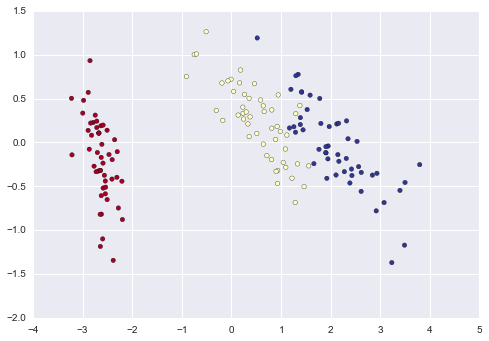In :
from sklearn.cluster import KMeans
k_means = KMeans(n_clusters=3, random_state=0) # Fixing the RNG in kmeans
k_means.fit(X)
y_pred = k_means.predict(X)

pl.scatter(X_reduced[:, 0], X_reduced[:, 1], c=y_pred,
cmap='RdYlBu');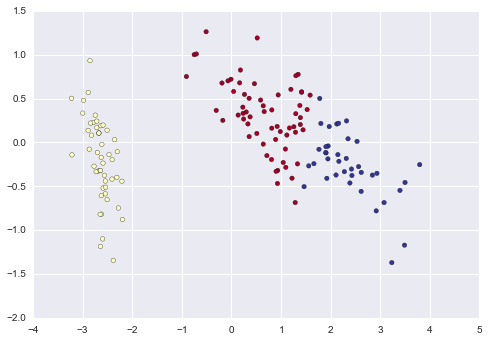K Means is an algorithm for unsupervised clustering: that is, finding clusters in data based on the data attributes alone (not the labels).

K Means is a relatively easy-to-understand algorithm. It searches for cluster centers which are the mean of the points within them, such that every point is closest to the cluster center it is assigned to.

Let's look at how KMeans operates on the simple clusters we looked at previously. To emphasize that this is unsupervised, we'll not plot the colors of the clusters:

In :
from sklearn.datasets.samples_generator import make_blobs
X, y = make_blobs(n_samples=300, centers=4,
random_state=0, cluster_std=0.60)
plt.scatter(X[:, 0], X[:, 1], s=50);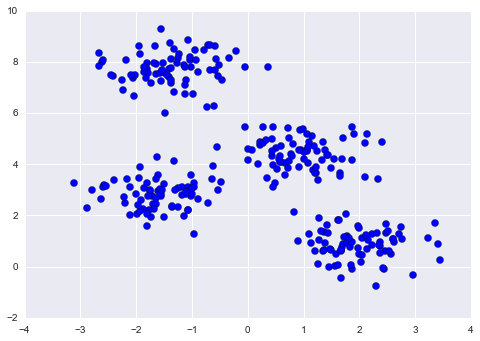By eye, it is relatively easy to pick out the four clusters. If you were to perform an exhaustive search for the different segmentations of the data, however, the search space would be exponential in the number of points. Fortunately, there is a well-known Expectation Maximization (EM) procedure which scikit-learn implements, so that KMeans can be solved relatively quickly.

In :
from sklearn.cluster import KMeans
est = KMeans(4)  # 4 clusters
est.fit(X)
y_kmeans = est.predict(X)
plt.scatter(X[:, 0], X[:, 1], c=y_kmeans, s=50, cmap='rainbow');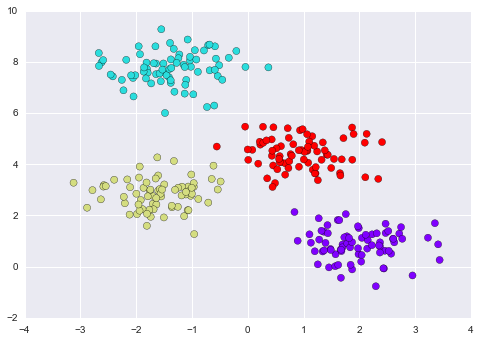The algorithm identifies the four clusters of points in a manner very similar to what we would do by eye!

## The K-Means Algorithm: Expectation Maximization¶

K-Means is an example of an algorithm which uses an Expectation-Maximization approach to arrive at the solution. Expectation-Maximization is a two-step approach which works as follows:

1. Guess some cluster centers
2. Repeat until converged A. Assign points to the nearest cluster center B. Set the cluster centers to the mean

Let's quickly visualize this process:

In :
from fig_code import plot_kmeans_interactive
plot_kmeans_interactive();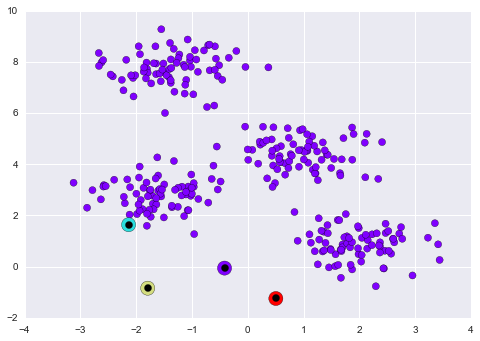This algorithm will (often) converge to the optimal cluster centers.

### KMeans Caveats¶

• The convergence of this algorithm is not guaranteed; for that reason, by default scikit-learn uses a large number of random initializations and finds the best results.

• The number of clusters must be set beforehand. There are other clustering algorithms for which this requirement may be lifted.